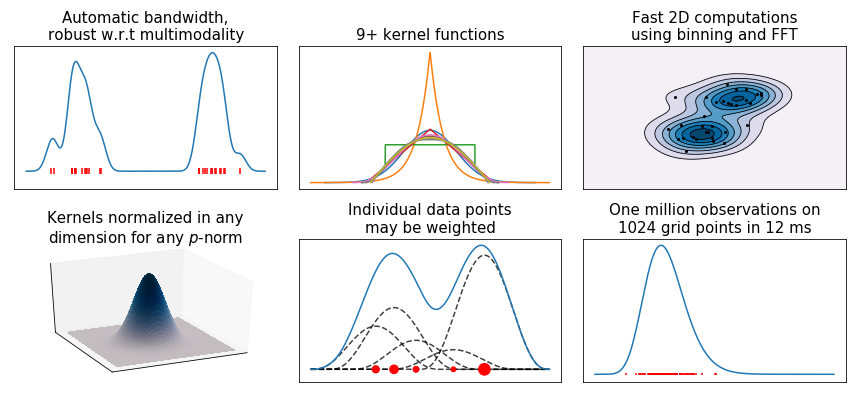# KDEpy Release 1.0.2

Kernel Density Estimation in Python.

Keywords
data-analysis, exploratory-data-analysis, kernel-density-estimation, python, python3, statistics
GPL-3.0-only
Install
``` pip install KDEpy==1.0.2 ```

# KDEpy

This Python 3.5+ package implements various kernel density estimators (KDE). Three algorithms are implemented through the same API: `NaiveKDE`, `TreeKDE` and `FFTKDE`. The class `FFTKDE` outperforms other popular implementations, see the comparison page.The code generating the above graph is found in examples.py.

## Installation

KDEpy is available through PyPI, and may be installed using `pip`:

``````pip install KDEpy
``````

If you have trouble on Ubuntu, try running `sudo apt install libpython3.X-dev`, where `3.X` is your Python version.

## Example code and documentation

Below is an example using NumPy as `np` and `scipy.stats.norm` to plot a density estimate. From the code below, it should be clear how to set the kernel, bandwidth (variance of the kernel) and weights. See the documentation for more examples.

```from KDEpy import FFTKDE
data = norm(loc=0, scale=1).rvs(2**3)
estimator = FFTKDE(kernel='gaussian', bw='silverman')
x, y = estimator.fit(data, weights=None).evaluate()
plt.plot(x, y, label='KDE estimate')```The package consists of three algorithms. Here's a brief explanation:

• `NaiveKDE` - A naive computation. Supports d-dimensional data, variable bandwidth, weighted data and many kernel functions. Very slow on large data sets.
• `TreeKDE` - A tree-based computation. Supports the same features as the naive algorithm, but is faster at the expense of small inaccuracy when using a kernel without finite support. Good for evaluation on non-uniform, arbitrary grids.
• `FFTKDE` - A very fast convolution-based computation. Supports weighted d-dimensional data and many kernels, but not variable bandwidth. Must be evaluated on an equidistant grid, the finer the grid the higher the accuracy. Data points may not be outside of the grid.

## Issues and contributing

### Issues

If you are having trouble using the package, please let me know by creating an Issue on GitHub and I'll get back to you.

### Contributing

Whatever your mathematical and Python background is, you are very welcome to contribute to KDEpy. To contribute, fork the project, create a branch and submit and Pull Request. Please follow these guidelines:

• Import as few external dependencies as possible.
• Use test driven development, have tests and docs for every method.
• Cite literature and implement recent methods.
• Unless it's a bottleneck computation, readability trumps speed.
• Employ object orientation, but resist the temptation to implement many methods -- stick to the basics.